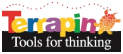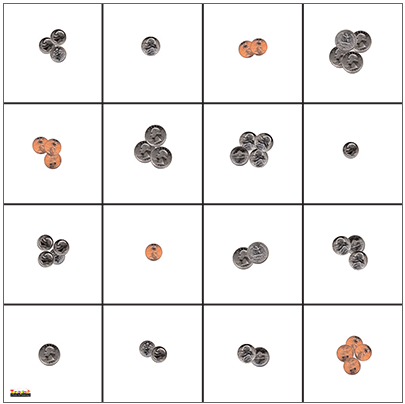Terrapin Resources

### US CoinsView a larger version of the mat.

The U.S. Coins Mat features images of American coins, including pennies, nickels, dimes, and quarters. Coins are pictured separately and also grouped in different quantities. The Coin Mat helps students learn identification, counting, and relative value as they move Bee-Bot from one square to another.

##### Lesson Ideas

Although these ideas refer to Bee-Bot, they can all be done with Blue-Bot as well.

Remind students to press X to erase the commands the previous student entered.

Lesson Idea 1: Learning Each Coin

• Divide students into groups.
• Name a coin and ask the first student to navigate Bee-Bot to that coin.
• The next student presses X to clear those commands.
• Repeat the process with the new student.

Lesson Idea 2: Going to Groups of Coins

• Divide students into groups.
• Name a group of coins, such as “Go to 4 dimes.”
• See if the student can go to the correct square.
• The next student presses X to clear those commands.
• Repeat the process with the new student.

Lesson Idea 3: Counting Money

• Divide students into groups.
• Name a group of coins, such as “Go to 4 dimes.”
• See if the student can go to the correct square and count how much money is shown there.
• The next student presses X to clear those commands.
• Repeat the process with the new student.

Lesson Idea 4: Using the Online Coin Mat

If students are using computers in your classroom, have them use the online Coin Mat in the Bee-Bot Emulator in a browser. They can explore the mat using these Lesson ideas.

Lesson Idea 5: Adding and Subtracting Money

• Divide students into groups.
• Have one student go to a square and tell how much money is there. Place a sticky note or blank card on that square.
• Have another student go to a different square and tell how much money is there.
• Have the group figure out what the total amount of money is (addition) and also which square shows more money, and how much more (subtraction).
##### Mat Details

This mat is incorporated into several of the Bee-Bot Lessons. These lessons give teachers lesson plans that are tied to national curriculum standards. They give students an opportunity to engage in over 180 learning activities using the floor Bee-Bot or Blue-Bot or the same activities in the online emulator.

The mat is made of washable vinyl and measures approximately 24” by 24” (60 cm by 60 cm)

View the mat in Terrapin’s store.